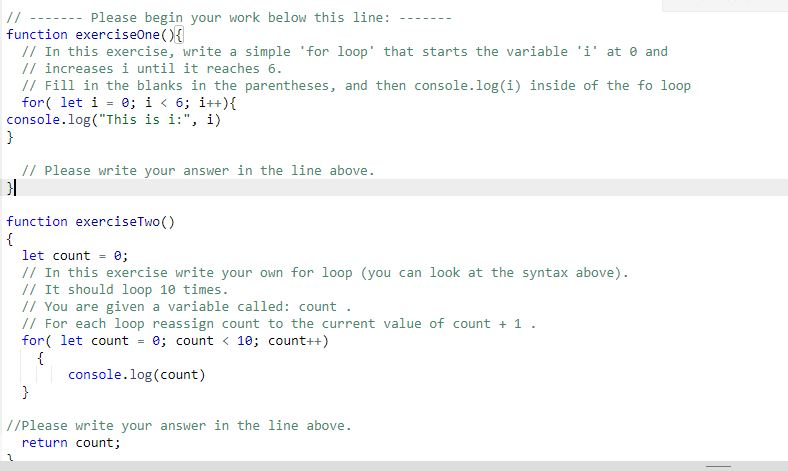Help

I'm doing an assignment 5 For Loops an I am stuck on the second question

This is what I wrote
function exerciseTwo()
{
let count = 0;
// In this exercise write your own for loop (you can look at the syntax above).
// It should loop 10 times.
// You are given a variable called: count .
// For each loop reassign count to the current value of count + 1 .
for( let count = 0; count < 10; count++)
{
console.log(count)
}

return count;
}You are viewing a single comment. View All
Coder100 (441)

Ok, maybe you should do

``````for(let count = 0; count <= 10; count++){
console.log(count);
}``````

So it fits the criteria still?
@cccpfsb